4WX Internet Search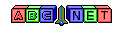Wet-bulb Temperature and Dewpoint Temperature from Air Temperature and Relative Humidity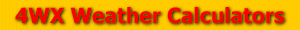# Wet-bulb Temperature and Dewpoint Temperature from Air Temperature and Relative Humidity

Note: This calculation is very complex and requires a knowledge of algebra.

From the user, an air temperature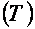, a relative humidity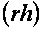, and a station pressure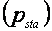are given. The air temperature must be converted to units of degrees Celsius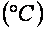, and the station pressure must be converted to units of millibars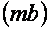or hectoPascals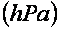.

To see how to convert temperatures and pressures, see the links below:

Then, the saturated vapor pressure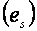can be calculated using the formula from the link below:

vaporPressure.php

Next, the equation for calculating relative humidity:can be solved for the dewpoint temperature:The dewpoint temperature will be in units of degrees Celsius. To see how to convert temperatures, see the link above.

Next, a wet-bulb temperature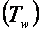must be calculated. The best way to do this is by using a Skew-T diagram which is used by the National Weather Service and other meteorologists for determining the current state of the atmosphere. A blank Skew-T diagram can be found here at this link:

For information on how to read and understand a Skew-T diagram, see the link below:

http://weather.unisys.com/upper_air/skew/details.php

For finding the wet-bulb temperature, first find the elevation of your location. Next, at the elevation of your location, plot the air temperature (in degrees Celsius) and the dewpoint temperature on the chart. Take the air temperature up the dry adiabat line and the dewpoint temperature up the theta line until they meet. At the point where they meet, come back down the moist (or wet) adiabat to the elevation of your station. This will be the wet-bulb temperature. For the Weather Calculator, a process of error checking is used to give an approximate wet-bulb temperature. Information on how the Weather Calculator approximates the wet-bulb temperature can found at this link here:

Back to 4WX Weather Calculators index page

4WX Calculators Credit and disclaimer